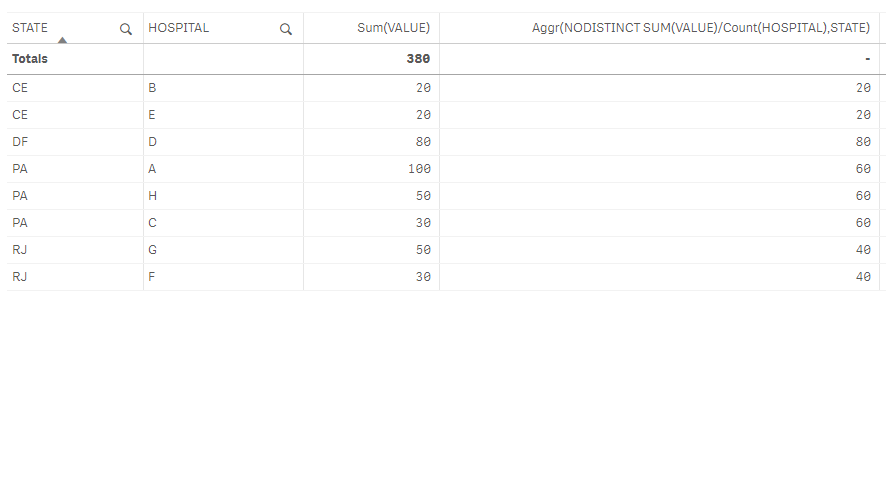# Qlik Sense App Development

cancel
Showing results for
Did you mean:
HighlightedContributor III

## Average, Aggregate and Sum - Calculating the Average by Dimension

Hi all,

I am having this problem calculating the Average of VALUE to each STATE.

The formula that I used is avg(aggr(sum(VALUE)/count(HOSPITAL),STATE)) as shown in the image.

So as you can see this work Ok for some rows (First occurrence of HOSPITAL) but for other rows I am getting Null (even that I just let "count(HOSPITAL)" instead of "sum(VALUE)/count(HOSPITAL)" ) I will get Null.

The goal is that each row has the average calculated correctly like others rows (values in the bottom table for each State).Someone know how can I solve this problem?I am attaching the QVF that I used, is just an example with an inline.

HOSPITAL,VALUE,STATE
A,100,PA
B,20,CE
C,30,PA
D,80,DF
E,20,CE
F,30,RJ
G,50,RJ
H,50,PA
];

I hope to learn a lot here and help if I can
Labels (10)

• ### sum()

1 Solution

Accepted Solutions
HighlightedContributor III

## Re: Average, Aggregate and Sum - Calculating the Average by Dimension

Hi,

I think you need the word NODISTINCT.

Aggr(NODISTINCT SUM(VALUE)/Count(HOSPITAL),STATE)